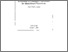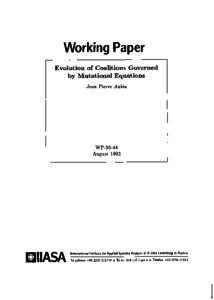Evolution of Coalitions Governed by Mutational Equations

Aubin J-P (1993). Evolution of Coalitions Governed by Mutational Equations. IIASA Working Paper. IIASA, Laxenburg, Austria: WP-93-044Preview
Text
WP-93-044.pdf

Abstract

In cooperative game theory as well as in some domains of economic regulation by shortages (queues or unemployment), one is confronted to the problem of evolution of coalitions of players or economic agents. Since coalitions are subsets and cannot be represented by vectors -- except if we embed subsets in the family of fuzzy sets, which are functions -- the need to adapt the theoy of differential equations and dynamical systems to govern the evolution of coalitions or subsets instead of vectors did emerge. Evolution of subsets (regarded as shapes or images) was also motivated by evolution equations of "tubes" in "visual servoing" on one hand, mathematical morphology on the other.

The "power spaces" in which coalitions, images, shapes, etc. evolve are metric spaces without a linear structure. However, one can extend the differential calculus to a mutational calculus for maps from one metric space to another, as we shall explain in this paper. The simple idea is to replace half-lines allowing to define difference quotients of maps and their various limits in the case of vector space by "transitions" with which we can also define differential quotients of a map. Their various limits are called "mutations" of a map. Many results of differential calculus do not really rely on the linear structure and can be adapted to the nonlinear case of metric spaces and exploited. Furthermore, the concept of differential equation can be extended to mutational equation governing the evolution in metric spaces. Basic Theorems as the Nagumo Theorem, the Cauchy-Lipschitz Theorem, the Center Manifold Theorem and the second Lyapunov Method hold true for mutational equations.

Item Type: Monograph (IIASA Working Paper) Dynamic Systems (DYN) IIASA Import 15 Jan 2016 02:02 13 Mar 2018 16:30 http://pure.iiasa.ac.at/3770View Item